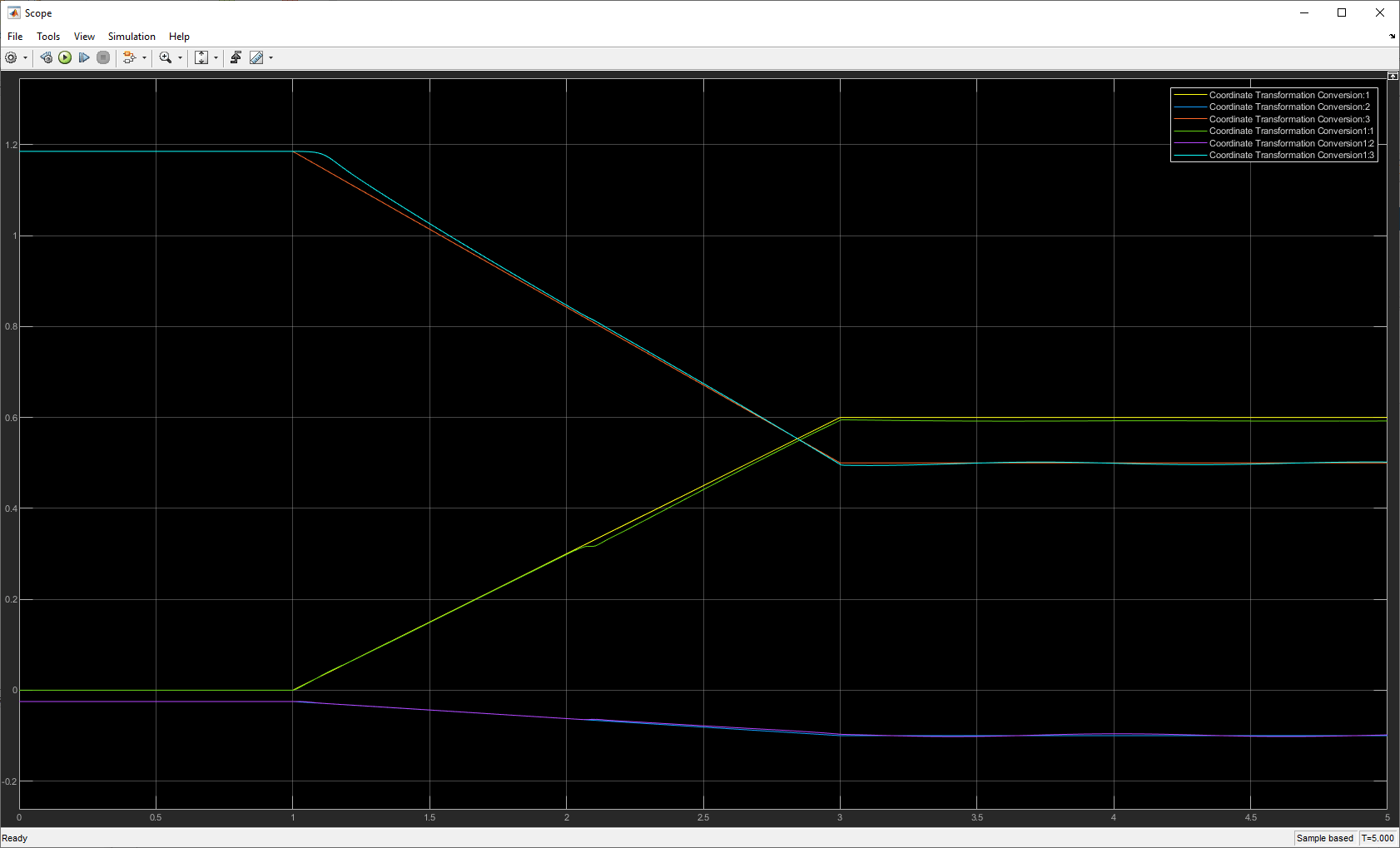This example shows how to use a Task Space Motion Model to follow a task space trajectory.

This example uses a Kinova Gen3 manipulator robot. Load the model using `loadrobot`.

```[gen3,metadata] = loadrobot("kinovaGen3",'DataFormat','column'); initialConfig = homeConfiguration(gen3); targetPosition = trvec2tform([0.6 -.1 0.5])```
```targetPosition = 4×4 1.0000 0 0 0.6000 0 1.0000 0 -0.1000 0 0 1.0000 0.5000 0 0 0 1.0000 ```

`open_system("followTaskSpaceTrajectoryModel.slx")`

### Trajectory Generation

The Transform Trajectory block creates a trajectory between the initial homogeneous transform matrix of the end effector of the Gen3, and the target position over a `3` second time interval.The Joint Space Motion Model uses a RigidBodyTree, `gen3`, to calculate the joint positions to follow the trajectory. The joint positions are converted to homogeneous transform matrices and then the converted to a translation vector so that it is easier to visualize.The joint target positions and the calculated joint values from the Task Space Motion Model connect to a Scope block. Using the legend, you can select a smaller set of signals to compare with better clarity. Observe that the `x`, `y`, and `z` positions of the end effector match closely with the `x`, `y`, and `z` positions of the trajectory to the target position.